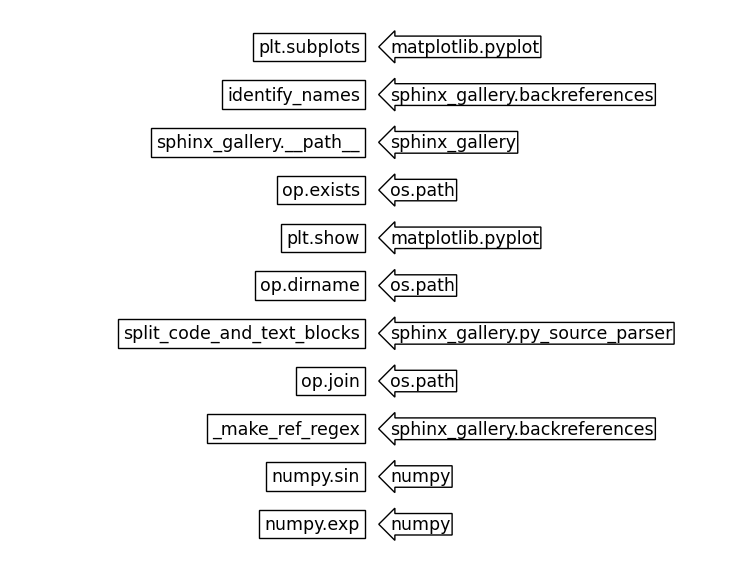# Identifying function names in a script#

This demonstrates how Sphinx-Gallery identifies when

1. a function/method/attribute/object is used or class instantiated in a code block

2. a function/method/attribute/object/class is referred to using sphinx markup in a text block.

Sphinx-Gallery examines both the executed code itself, as well as the text blocks (such as this one, or the one below) for these references and identifies the module they belong to. This means that by writing `numpy.sin` and `numpy.exp` here, they will be identified even though they are not used in the code. This is useful in particular when functions return classes (meaning it is not explicitly instantiated) – if you add them to the documented blocks of examples that use them, they will be added to backreferences.

```# Code source: Óscar Nájera

import os.path as op  # noqa, analysis:ignore
import matplotlib.pyplot as plt
import sphinx_gallery
from sphinx_gallery.backreferences import identify_names, _make_ref_regex
from sphinx_gallery.py_source_parser import split_code_and_text_blocks

filename = "plot_6_function_identifier.py"
if not op.exists(filename):
filename = (
__file__
if "__file__" in locals()
else op.join(op.dirname(sphinx_gallery.__path__), "examples", filename)
)

_, script_blocks = split_code_and_text_blocks(filename)

names = identify_names(script_blocks, _make_ref_regex())
```

In the code block above, we use the internal function `identify_names` to obtain all identified names from this file and their full resolved import path. We then plot this below, where the identified names functions are on the left and the full resolved import path is on the right.

```fontsize = 12.5
figheight = 3 * len(names) * fontsize / 72
fig, ax = plt.subplots(figsize=(7.5, figheight))
ax.set_visible(False)

for i, (name, obj) in enumerate(names.items(), 1):
fig.text(
0.48,
1 - i / (len(names) + 1),
name,
ha="right",
va="center",
size=fontsize,
transform=fig.transFigure,
bbox=dict(boxstyle="square", fc="w", ec="k"),
)
fig.text(
0.52,
1 - i / (len(names) + 1),
obj["module"],
ha="left",
va="center",
size=fontsize,
transform=fig.transFigure,